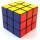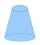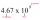# Volume + real numbers - math problems

#### Number of problems found: 15

• CubesCube, which consists of 8 small cubes with edge 3 dm has volume:
• Cube zoomHow many percents do we increase the volume and surface of the cube if we magnify its edge by 38 %?
• 2x coneCircular cone height 84 cm was cut plane parallel with base. Volume of these two small cones is the same. Calculate the height of the smaller cone.
• Spherical capFrom the sphere of radius 11 was truncated spherical cap. Its height is 6. What part of the volume is a spherical cap from the whole sphere?
• CylindersThe area of the side of two cylinders is the same rectangle of 33 mm × 18 mm. Which cylinder has a larger volume and by how much?
• Axial sectionThe axial section of the cone is an equilateral triangle with area 168 cm2. Calculate the volume of the cone.
• HoleIn the center of the cube with edge 14 cm we will drill cylinder shape hole. Volume of the hole must be 27% of the cube. What drill diameter should be chosen?
• Cube in a sphereThe cube is inscribed in a sphere with a volume 7253 cm3. Determine the length of the edges of a cube.
• RotationThe right triangle with legs 11 cm and 18 cm rotate around the longer leg. Calculate the volume and surface area of the formed cone.
• PumpWhat power has a pump output to move 4853 hl of water to a height of 31 m for 8 hours?
• Cone A2VThe surface of the cone in the plane is a circular arc with central angle of 126° and area 415 cm2. Calculate the volume of a cone.
• Copper windingCalculate the current flowing through the copper winding at an operating temperature of 70°C. Celsius, if the winding diameter is 1.128 mm and the winding length is 40 m. The winding is connected to 8V.
• CubesOne cube is an inscribed sphere and the other one described. Calculate the difference of volumes of cubes, if the difference of surfaces in 257 mm2.
• Scientific notationApproximately 7.5x105 gallons of water flow over a waterfall each second. There are 8.6x104 seconds in 1 day. Select the approximate number of gallons of water that flow over the waterfall in 1 day.
• PoolIf water flows into the pool by two inlets, fill the whole for 19 hours. The first inlet filled pool 5 hour longer than the second. How long pool take to fill with two inlets separately?

Do you have an interesting mathematical word problem that you can't solve it? Submit a math problem, and we can try to solve it.

We will send a solution to your e-mail address. Solved examples are also published here. Please enter the e-mail correctly and check whether you don't have a full mailbox.

Please do not submit problems from current active competitions such as Mathematical Olympiad, correspondence seminars etc...

Tip: Our volume units converter will help you with the conversion of volume units. Volume - math problems. Real numbers - math problems.